Next: *ELECTRICAL CONDUCTIVITY Up: Input deck format Previous: *DYNAMIC   Contents

## *ELASTIC

Keyword type: model definition, material

This option is used to define the elastic properties of a material. There is one optional parameter TYPE. Default is TYPE=ISO, other values are TYPE=ORTHO and TYPE=ENGINEERING CONSTANTS for orthotropic materials and TYPE=ANISO for anisotropic materials. All constants may be temperature dependent. For orthotropic and fully anisotropic materials, the coefficients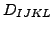satisfy the equation: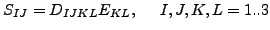(471)

where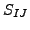is the second Piola-Kirchhoff stress and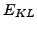is the Lagrange deformation tensor (nine terms on the right hand side for each equation). For linear calculations, these reduce to the generic stress and strain tensors.

An isotropic material can be defined as an anisotropic material by defining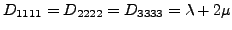,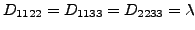and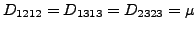, where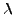and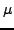are the Lamé constants .

First line:

• *ELASTIC
• Enter the TYPE parameter and its value, if needed

Following line for TYPE=ISO:

• Young's modulus.
• Poisson's ratio.
• Temperature.
Repeat this line if needed to define complete temperature dependence.

Following lines, in a pair, for TYPE=ORTHO: First line of pair:

•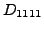.
•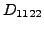.
•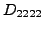.
•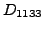.
•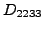.
•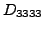.
•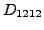.
•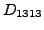.
Second line of pair:
•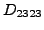.
• Temperature.
Repeat this pair if needed to define complete temperature dependence.

Following lines, in a pair, for TYPE=ENGINEERING CONSTANTS: First line of pair:

•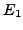.
•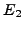.
•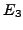.
•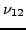.
•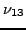.
•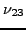.
•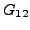.
•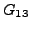.
Second line of pair:
•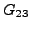.
• Temperature.
Repeat this pair if needed to define complete temperature dependence.

Following lines, in sets of 3, for TYPE=ANISO: First line of set:

•.
•.
•.
•.
•.
•.
•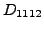.
•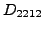.
Second line of set:
•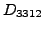.
•.
•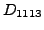.
•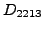.
•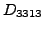.
•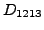.
•.
•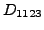.
Third line of set:
•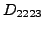.
•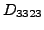.
•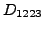.
•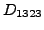.
•.
• Temperature.
Repeat this set if needed to define complete temperature dependence.

Example:

*ELASTIC,TYPE=ORTHO
500000.,157200.,400000.,157200.,157200.,300000.,126200.,126200.,
126200.,294.


defines an orthotropic material for temperature T=294. Since the definition includes values for only one temperature, they are valid for all temperatures.

Example files: aniso, beampo1.Next: *ELECTRICAL CONDUCTIVITY Up: Input deck format Previous: *DYNAMIC   Contents
guido dhondt 2018-12-15